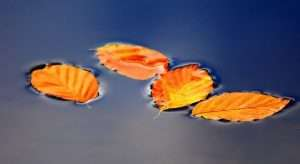Join Our WhatsApp Group!

# Definition of Surface Tension – Formula, Example & Effect

## Surface Tension

It is defined as the force per unit length acting at right angles to an imaginary line drawn on the free surface of the liquid. When the surface is between two liquids (such as water and oil), it is known as “interface tension”.T = F/L

T = Surface Tension

F = Surface Force

L = Total length

### Units of Surface Tension

• I. The unit of surface tension is Newton/meter.
• CGS unit of surface tension is dyn/cm.
• Work was done in forming a bubble inside a liquid,

W = T x 4πr2

Where T is free surface energy density equal to W/dA

• Work was done in forming a bubble in the air

w = T x (2×4πr2)

= 8πr2T

• Work was done in spraying a liquid drop of radius R into equal droplets

(w = T x 4πR2 x (N1/3-1)

## What is Surface Tension?

It is a phenomenon in which the surface of a liquid, where the liquid is in contact with the gas, acts like a thin elastic sheet. The term is typically used if the liquid surface is in contact with the gas.### Cause of Surface Tension

The different intermolecular forces, like Van der Waals forces, draw the liquid particles together. Along the surface, the particles are pulled towards the rest of the liquid.

### Effect of Surface Tension

1. #### Effect of Impurity and Chemical

By adding impurities to the liquid, the nature of the bonding between the molecules changes.

Example: When common salt is dissolved in water, the surface tension of the saltwater is more as compared to water.

Example: The addition of detergents, soap as well as phenol decreases the surface tension of water.

1. #### Effect of Temperature

The surface tension of liquid decreases by increasing temperature. If the temperature increases, the average separation b/w the molecules increases & due to that the cohesive force decreases.

The surface tension of liquid becomes zero (0) at a particular temperature known as the critical temperature of that liquid.

### Application of Surface Tension

• Paste in toothpaste spreads more freely in the mouth because it contains soap which reduces surface tension.
• Mosquito eggs float on the surface of water due to surface tension of water. When kerosene is sprayed on the surface of the water, the surface tension gets lowered and eggs go down inside the water.
• If detergent is added in water, the surface tension of the resulting soap solution is less than water. This increases the area of contact and is the reason for the cleaning ability of the soap solution.

### Example of Surface Tension

#### Insects walking on water

Insects are able to walk on water, such as the water strider.  Legs of insects are formed to distribute their weight, causing the surface of the liquid to become depressed, minimizing the potential energy to create a balance of forces so that the spider car move across the surface of the water without breaking through the surface.

#### Drop of water

When using a water dropper the water does not flow in a continuous stream, but rather in a series of drops. The spherical shape of the drops is caused by the surface tension of the water. However, the only reason the drop of water is not completely spherical is because of the force of gravity pulling down on it. In the absence of gravity, the drop would minimize the surface area in order to minimize surface tension, which would result in a perfectly spherical shape.

It is because of surface tension, most of the fruits we see are spherical or roughly spherical in shape. They intend to occupy the minimum surface area for the volume of matter, they contain.

#### Soap Bubble

When we blow a soap bubble, we create a pressurized bubble of air that is contained within a thin, elastic surface of the liquid. Most liquids cannot maintain a stable surface tension to create a bubble that is why soap is generally used in the process, it stabilizes the surface tension through something called the Marangoni effect.

If the bubble is blown, the surface film tends to contract. This causes the pressure inside the bubble to increase. The size of the bubble stabilizes at a where gas inside the bubble won’t contract any further, at least without popping the bubble.

#### Needle floating on water

The density of these objects is greater than water yet the surface tension along the depression is enough to counteract the force of gravity pulling down on the metal object. Floating of eggs in water is also because of surface tension.

## The pressure inside a Soap Bubble

Suppose pressure inside the soap bubble of radius R, ignoring the very slight difference in inner and outer radius, the circumference is 2πR.

Each inner and outer surface will have a pressure of r along the entire length, so that total force from the surface tension.

2r = 2πR

Inside the bubble, there is a pressure ‘p’ that acts over the entire cross-section πR2, resulting in a total force of R2).

The bubble is stable, the sum of these forces must be zero.

2r(2πR) = p(pπR2)

OR

p = 4r/R

## Pressure in a liquid drop

Analyzing a drop of liquid, as opposed to a soap bubble, is simpler. Instead of two surfaces, there is only the exterior surface to consider, so a factor of 2 drops out of the earlier equation to yield:

p – pe = 2r/R